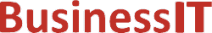O nás     Inzerce     KontaktSpolehlivé informace o IT již od roku 2011[Linux manuál]

# logb, logbf, logbl: získat exponent plovoucí desetinné čárky

Originální popis anglicky: logb, logbf, logbl - get exponent of a floating point value

Návod, kniha: Linux Programmer's Manual

## STRUČNĚ

#include <math.h>

double logb(double x);

float logbf(float x);

long double logbl(long double x);

## POPIS / INSTRUKCE

These functions extract the exponent of x and return it as a floating-point value. If FLT_RADIX is two, logb(x) is equal to floor(log2(x)), except it's probably faster.
If x is de-normalized, logb() returns the exponent x would have if it were normalized.
If x is zero, -HUGE_VAL (resp. -HUGE_VALF, -HUGE_VALL) is returned, and a pole error occurs. If x is infinite, plus infinity is returned. If x is NaN, NaN is returned.

## CHYBY / ERRORY

In order to check for errors, set errno to zero and call feclearexcept(FE_ALL_EXCEPT) before calling these functions. On return, if errno is non-zero or fetestexcept(FE_INVALID | FE_DIVBYZERO | FE_OVERFLOW | FE_UNDERFLOW) is non-zero, an error has occurred.
If an error occurs and (math_errhandling & MATH_ERRNO) is non-zero, then errno is set to ERANGE. If an error occurs and (math_errhandling & MATH_ERREXCEPT) is non-zero, then the divide-by-zero floating-point exception is raised.
A pole error occurs when x is zero.

## HISTORY

The logb function occurs in BSD 4.3.

ISO C99

## SOUVISEJÍCÍ

log(3), ilogb(3)
 2004-10-31
©2011-2020 BusinessIT.cz, ISSN 1805-0522 | Názvy použité v textech mohou být ochrannými známkami příslušných vlastníků.
Provozovatel: Bispiral, s.r.o., kontakt: BusinessIT(at)Bispiral.com | Inzerce: Best Online Media, s.r.o., zuzana@online-media.cz
O vydavateli | Pravidla webu BusinessIT.cz a ochrana soukromí | pg(9305)